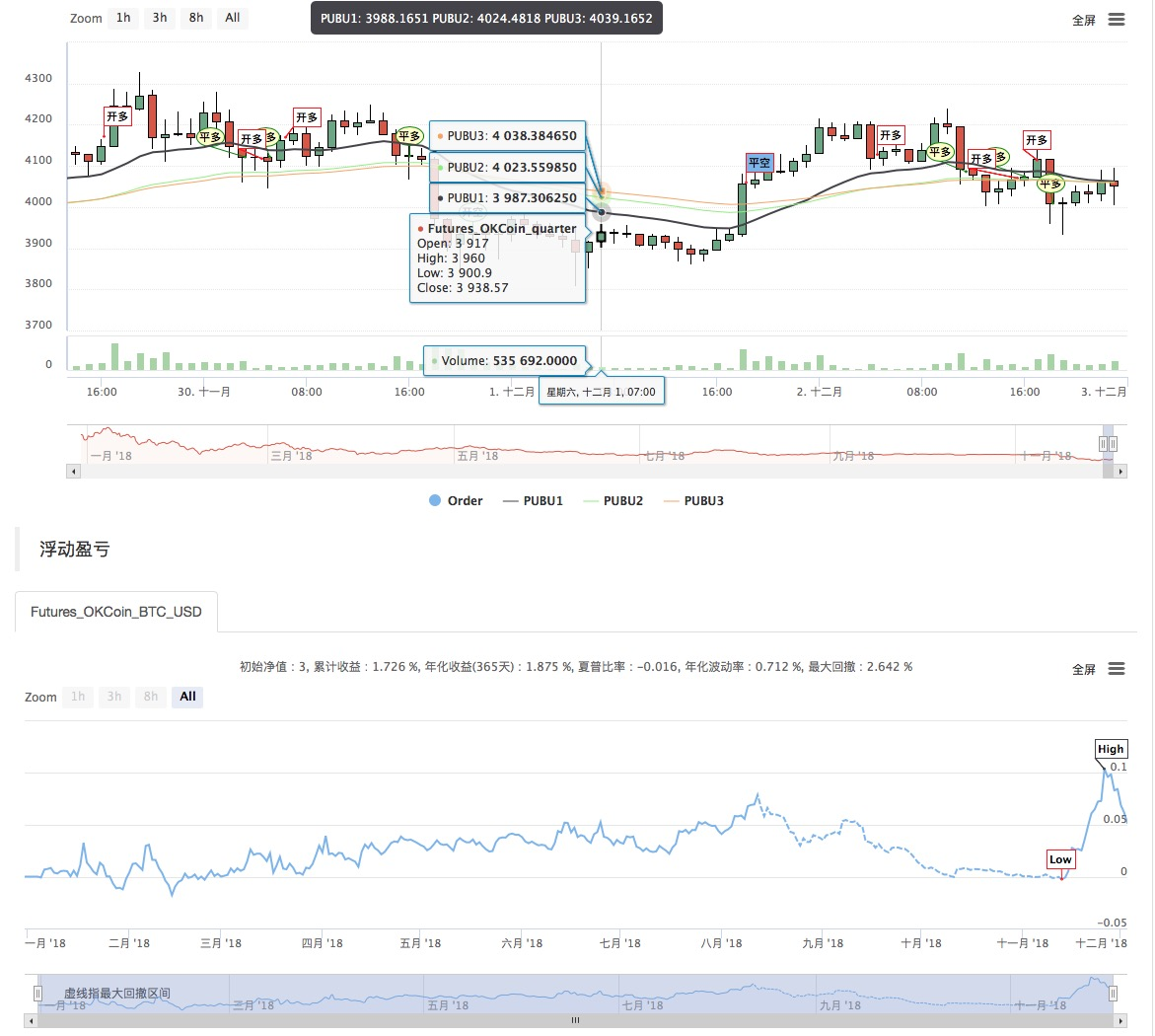# 瀑布线交易策略在发明者量化平台的实现与应用

Author: , Created: 2019-07-29 15:22:39, Updated: 2019-07-30 10:30:20

## 实现一个瀑布线策略

• 策略名称：瀑布线PUBU交易策略
• 数据周期：15M
• 支持：商品期货，数字货币• 主图
``````瀑布线1，公式：PUBU1^^(EMA(C,N1)+EMA(C,N12)+EMA(C,N14))/3;

``````

My语言源码：

``````// 指标
PUBU1^^(EMA(C,N1)+EMA(C,N1*2)+EMA(C,N1*4))/3;
PUBU2^^(EMA(C,N2)+EMA(C,N2*2)+EMA(C,N2*4))/3;
PUBU3^^(EMA(C,N3)+EMA(C,N3*2)+EMA(C,N3*4))/3;

BKVOL=0 AND BARPOS>N3 AND C>PUBU1 AND PUBU1>PUBU2 AND PUBU2>PUBU3,BPK;
SKVOL=0 AND BARPOS>N3 AND C<PUBU1 AND PUBU1<PUBU2 AND PUBU2<PUBU3,SPK;

C<PUBU3,SP(BKVOL);
C>PUBU3,BP(SKVOL);
C<PUBU2 AND PUBU1<PUBU2 AND C>BKPRICE,SP(BKVOL);
C>PUBU2 AND PUBU1>PUBU2 AND C<SKPRICE,BP(SKVOL);
AUTOFILTER;
``````

More